算法之"高手过招"[最小K个数]1.1 最小K个数

• **难度系数: ☆☆☆☆

• 题目来源: LeetCode 下分治策略专题

• 题目描述: 设计一个算法, 找出数组中最小的k个数. 以任意顺序返回这k个数均可;

• 输入:arr  =  [1,3,5,7,2,4,6,8] , k = 4;

• 输出: [1,2,3,4]

• 提示:

• 0 <= len(arr) <= 100000

• 0 <= k <= min(100000, len(arr))

• 题目解读:

• 这个问题就是想要你从10w 个数字找出 最小的k 个数;

• 关键词:  数量级10w, 最小的k个数 , 返回顺序任意;

• 出现过企业面试题: 字节跳动,腾讯

1.2 快排实现最小K个数

LeetCode 执行结果问题分析: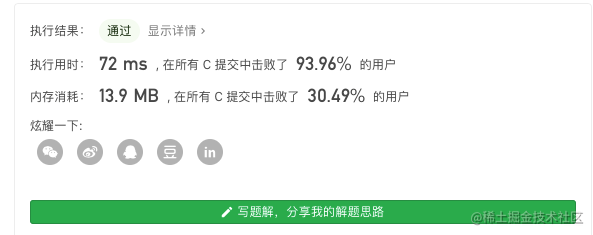1.3 快速排序思想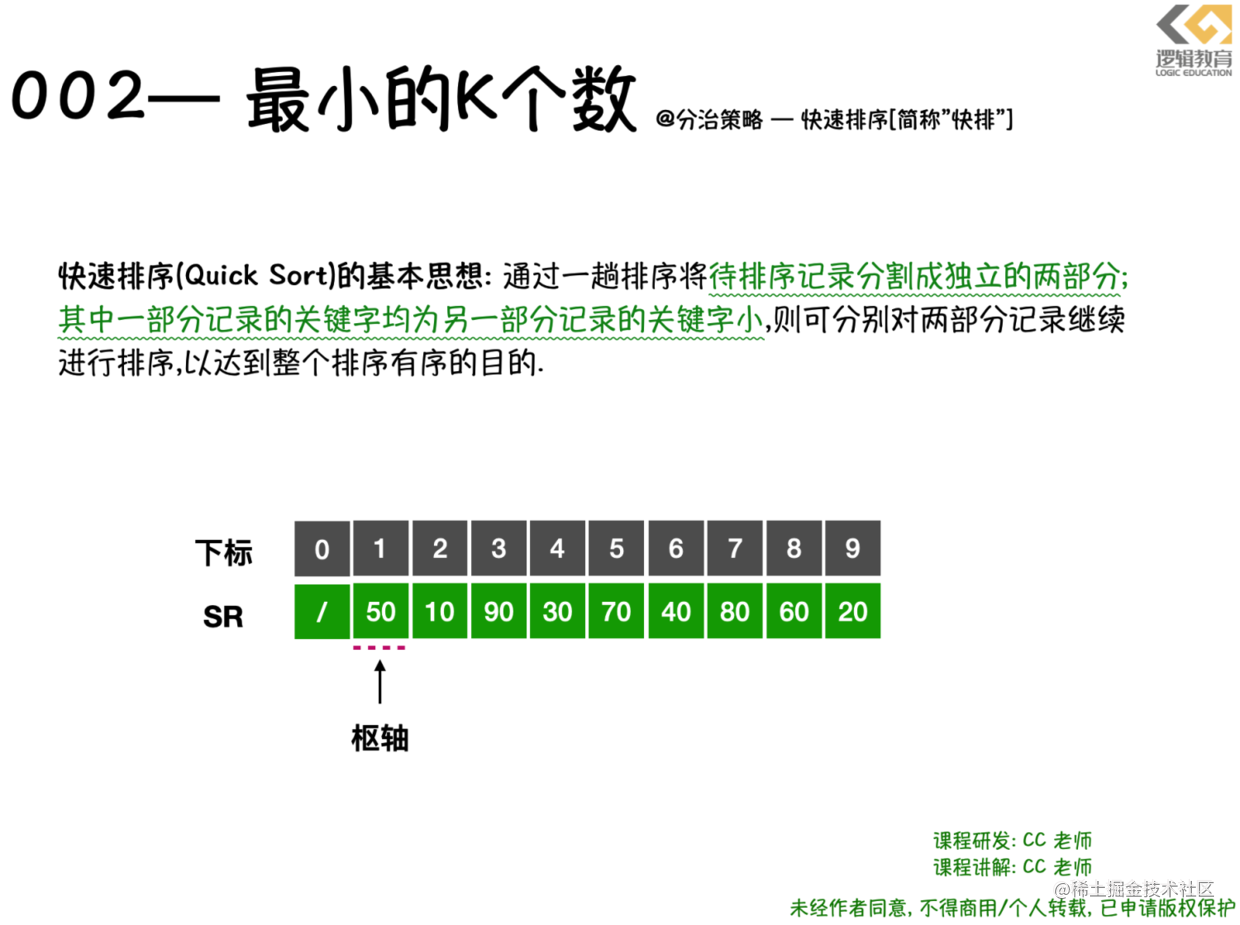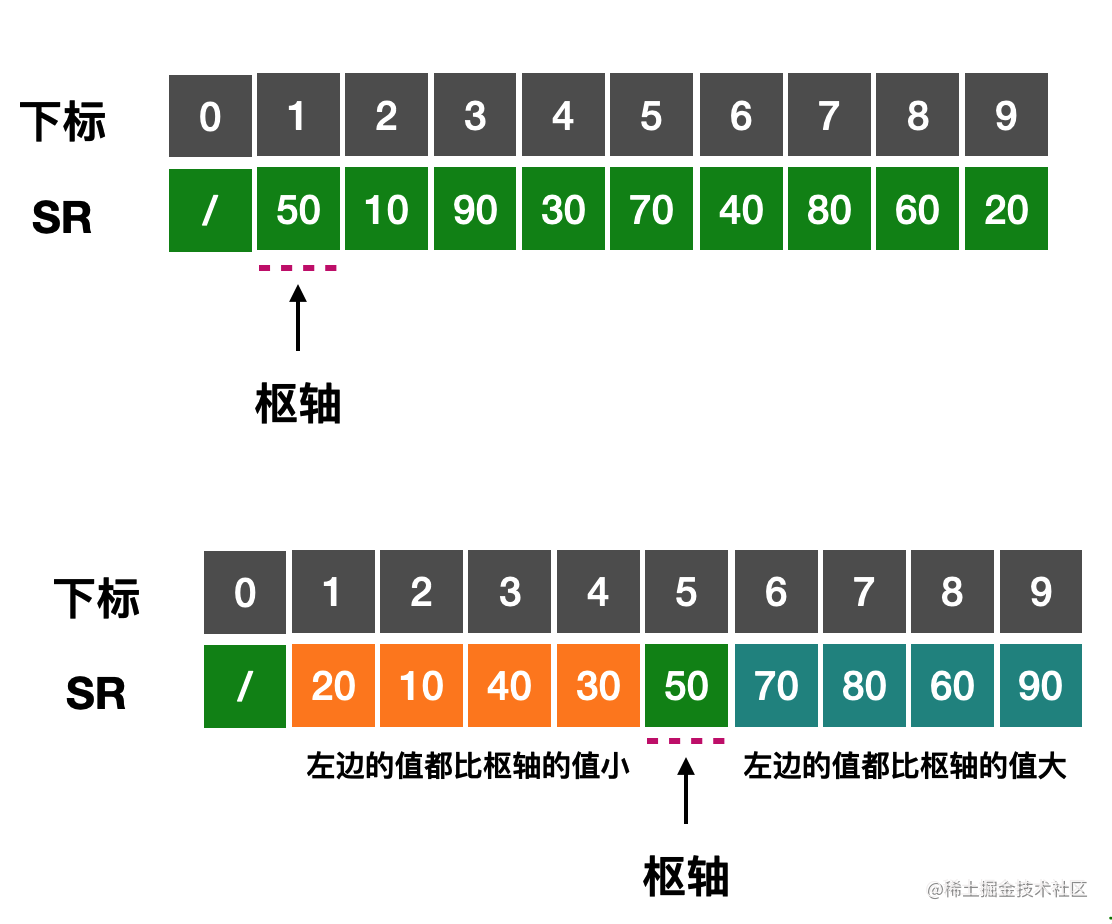• 判断当前的数组是否为空/数组长度是否小于0,以及查找的k数是否小于0,返回的size是否赋予了对应的地址空间;
• low = 0, hight = arrSize -1;
• 求得枢轴,并且将数组枢轴左边的关键字都比它小, 右边的关键字都比枢轴对应的关键字大;
• 将数组一分为二,对低子表进行排序,对高子表进行排序;
• 排序结束后,将数组arr 中前k个数据存储到 ans 数组中并返回;

**那么我们来看看 **smallestK 的代码实现:

void QSort(int *arr, int low, int hight){
int pivot ;
if (low < hight) {
//将L->r[low,high]一分为二,算出中枢轴值 pivot;
pivot = Partition2(arr, low, hight);

printf("arr[%d] = %d\n",pivot,arr[pivot]);
//对低子表递归排序;
QSort(arr, low, pivot-1);
//对高子表递归排序
QSort(arr, pivot+1, hight);
}
}

int* smallestK2(int* arr, int arrSize, int k, int* returnSize){
//1.判断数组是否为空,且arrsize 小于0则不符合排序的前提;
//1.判断寻找最小的K数,且returnSize 空间是否开辟成功,不符合则返回Null
if(arr == NULL || arrSize <= 0 || k <= 0 || returnSize == NULL){
if(returnSize != NULL) *returnSize = 0;
return NULL;
}

//进行快速排序QSort
QSort(arr, 0, arrSize);

//4. 创建一个数组reslut, 数组长度为k;
int* reslutArr = malloc(sizeof(int) * k);
*returnSize = k;
//循环将排序后的arr数组中的前k个元素存储到数组reslutArr 中;
for(int i = 0; i < k; i++){
reslutArr[i] = arr[i];
}

return reslutArr;
}

• QSort (L,1,L->length) 中的1L->Length代码的意思,其实就是对当前待排序的最小下标值low和最大下标值high.
• 这段代码的核心就是求解枢轴;pivot = Partition(L,low,high). 在执行之前,L.r数组值为{50,10,90,30,70,40,80,60,20}.
• Partition 函数要做的,就先选取当中一个关键字.比如选择第一个关键字50. 然把它放在一个位置上,使得它左边的值都比它小, 右边的值都比它大. 我将这样的关键字称为枢轴(pivot);
• 经过Partition(L,1,9)之后,数组变成了 {20,10,40,30,50,70,80,60,90}; 并返回了5给pivot. 数字5表示50放置在数组下标为5的位置.
• 此时把原来位于50左右的2个数组{20,10,40,30}{70,80,60,90}.
• 后面的递归就是调用 QSort(L,1,5-1),QSort(L,5+1,9). 其实就是对{20,10,40,30}{70,80,60,90}. 分别同样进行Partition 操作,直到顺序全部正确为止;

Partition2函数的功能

1. 选取当中一个关键字作为枢轴;
2. 将它放在一个合适的位置上, 使得它的左边的值都比它小, 右边的值都比它大;

1.4 Partition 函数的实现与分析

1. 那么如何寻找枢轴变量?
2. 如果将枢轴变量放在合适的位置,并且使得左侧关键字均比它小,且右侧的均比它大;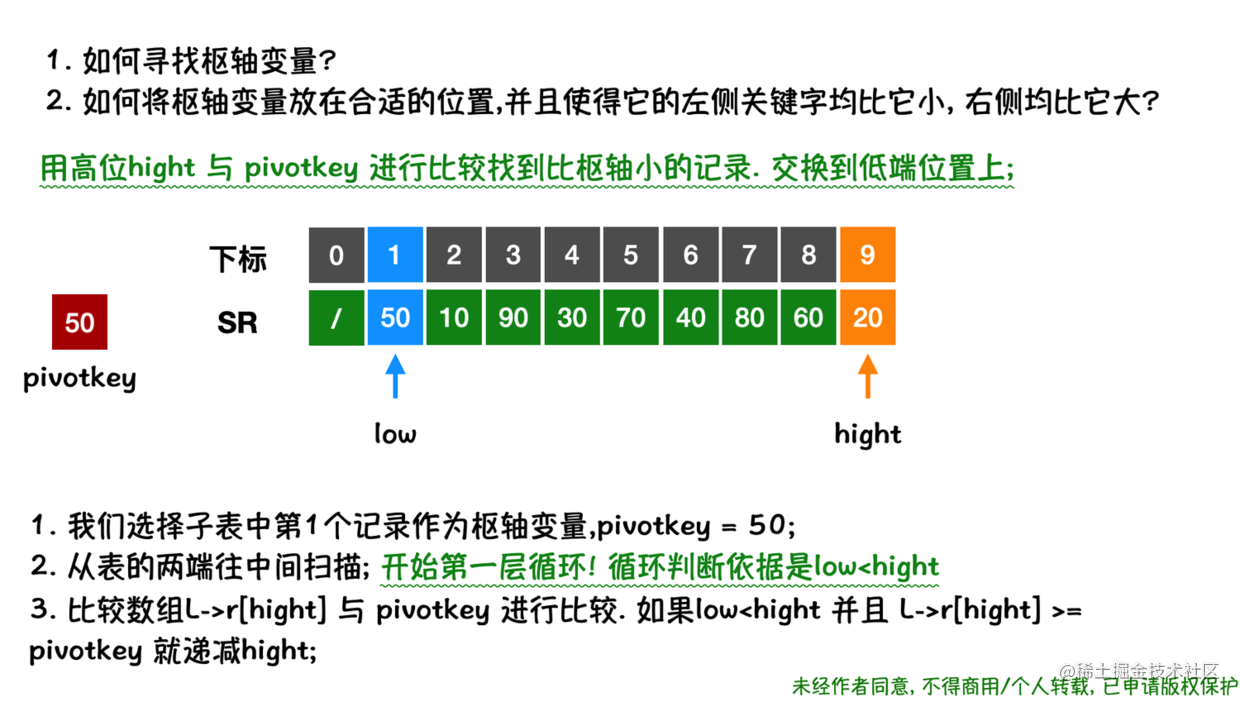• 我们选择子表中第1个记录作为枢轴变量,pivotkey = 50;
• 从表的两端往中间扫描; 开始第1层循环! 循环判断依据是low<high
• 用高位high 与 pivotkey 进行比较找到比枢轴小的记录. 交换到低端位置上;
• 比较数组L->r[high] 与 pivotkey 进行比较. 如果low<hight 并且 L->r[high] >= pivotkey 就递减high;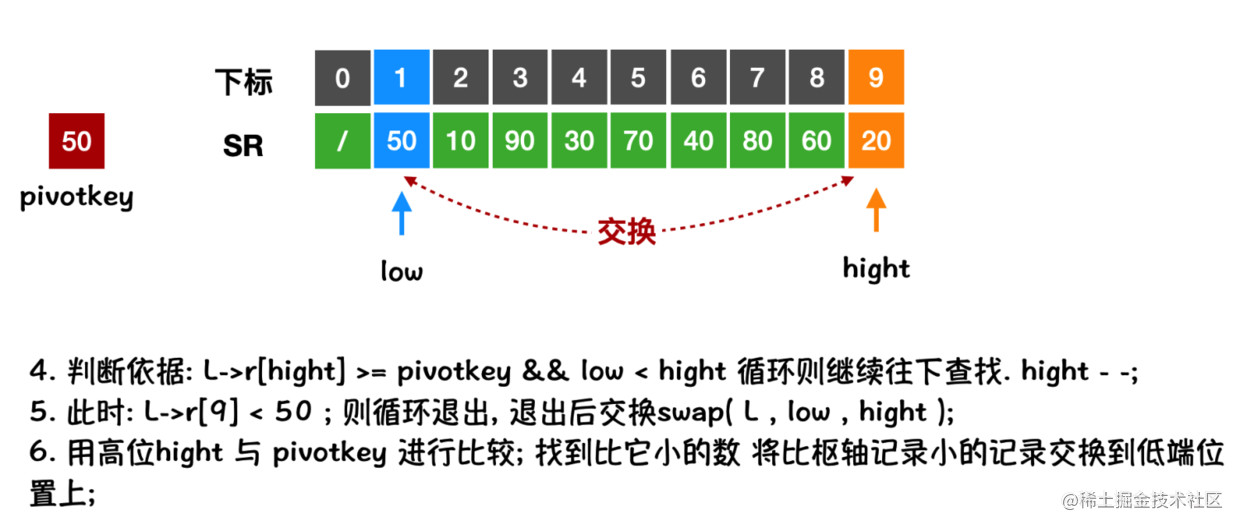• 判断依据: L->r[high] >= pivotkey && low < high 循环则继续往下查找. high 递减;
• 此时, 如图. 当high = 9, low = 1; L->r = 20; L->r = 50; 所以不满足循环条件,退出循环, 那么此时需要交换Swap(L,1,9); 使得比pivotkey 小的数据,交换到低端位置上;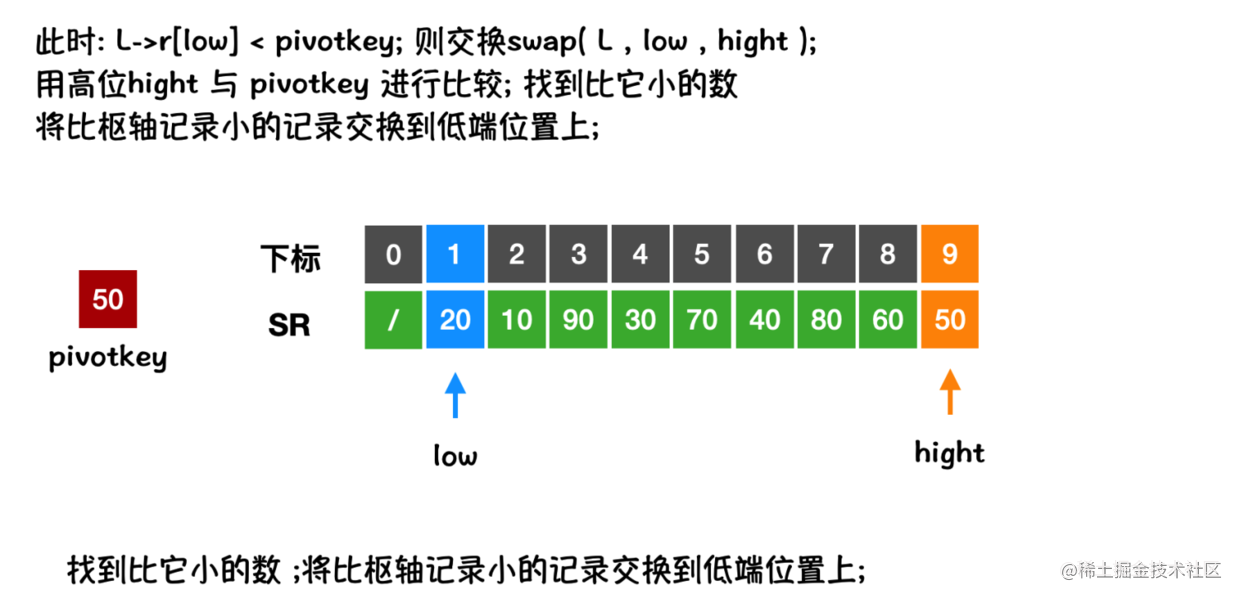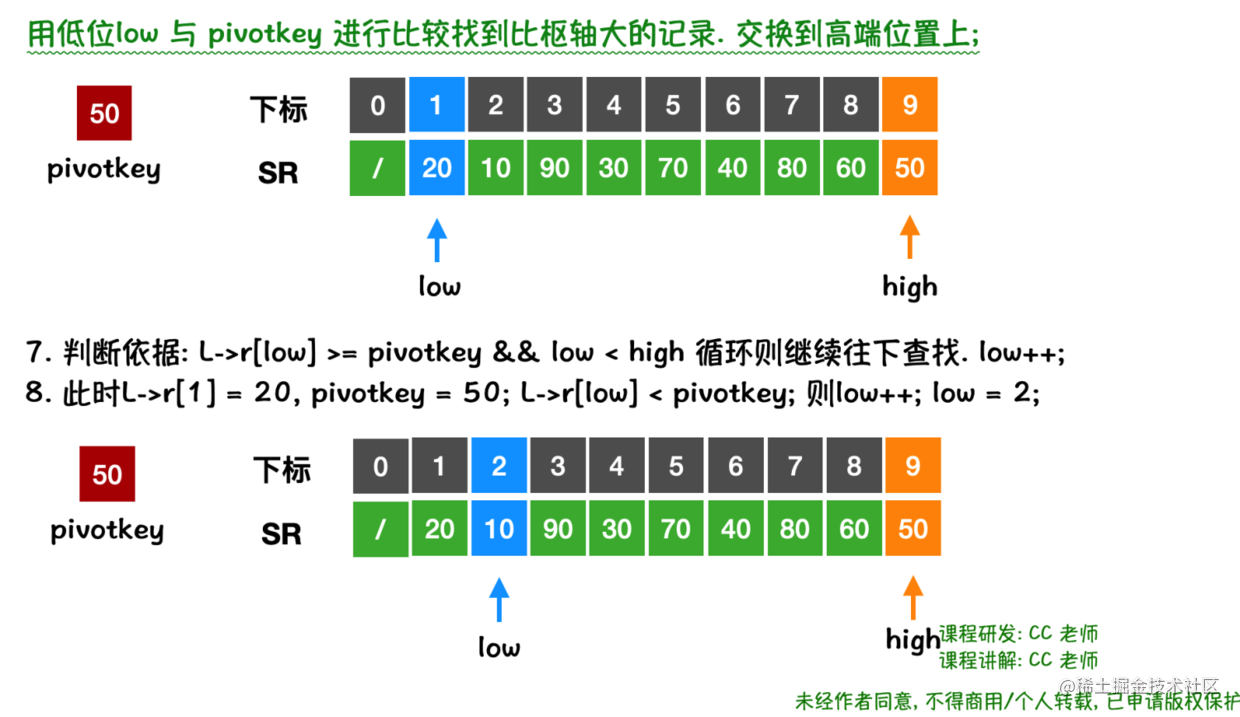• 接下来, 用低位low 与 pivotkey 进行比较找到比枢轴大的记录. 交换到高端位置上;
• 判断依据: L->r[low] >= pivotkey && low < high循环则继续往下查找. low++;
• 此时,L->r = 20, pivotkey = 50; L->r[low] < pivotkey; 则low++; low = 2;

• 此时L->r = 10, pivotkey = 50; L->r[low] < pivotkey; 则low++; low = 3;
• 此时L->r = 90, pivotkey = 50; L->r[low] > pivotkey; 则循环退出.
• 交换L->r[low] 与 L->r[high]的值; swap(L ,3,9);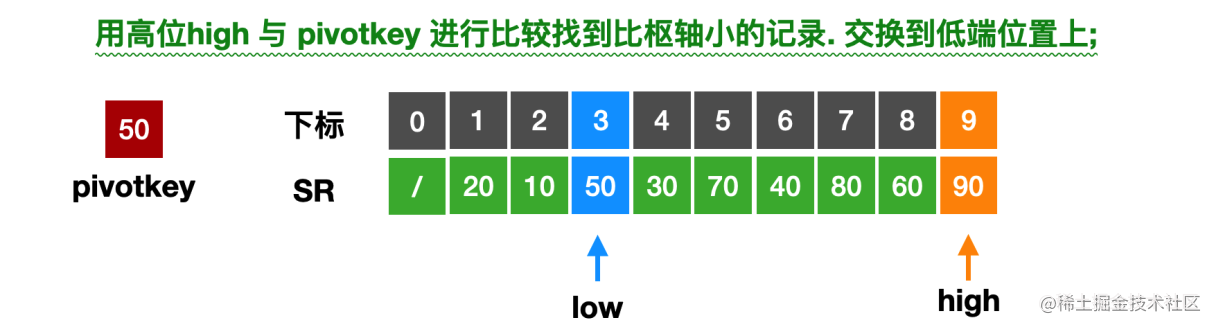• 第1次 往中间扫描结束!
• 但是此时low < high . low = 3,high = 9. 还可以继续进行第2次 往中间两端交替向中间扫描;

Partition 函数的思路:

• 选取第一个关键字作为枢轴;

• 只要(low < high) 就循环持续的将表的两端进行交替向中间扫描 (****两端交替循环)

• while 遍历从[low,high]的高端位置开始找,找到比枢轴小的关键字(高位调整循环)

• 如没有找到,则修改范围. 将high 递减;

• 如果找到进行交换到低端位置 swap(L,low,high);

• while 遍历从[low,high]的低端位置开始找,找到比枢轴大的关键字(低位调整循环)

• 如果没有找到,则修改范围,将low 递增;

• 如果找到进行交换到高端位置 swap(L,low,high);

Partition 代码实现:

//③交换顺序表L中子表的记录，使枢轴记录到位，并返回其所在位置
//此时在它之前(后)的记录均不大(小)于它
int Partition(SqList *L,int low,int high){
int pivokey;
//pivokey 保存子表中第1个记录作为枢轴记录;
pivokey = L->r[low];
//① 从表的两端交替地向中间扫描;
while (low < high) {

//② 比较,从高位开始,找到比pivokey更小的值的下标位置;
while (low < high &&  L->r[high] >= pivokey)
high--;
//③ 将比枢轴值小的记录交换到低端;
swap(L, low, high);
//④ 比较,从低位开始,找到比pivokey更大的值的下标位置;
while (low < high && L->r[low] <= pivokey)
low++;
//⑤ 将比枢轴值大的记录交换到高端;
swap(L, low, high);

}

//返回枢轴pivokey 所在位置;
return low;
}

最小K个数[快速排序实现策略]完整代码实现

int Partition2(int *L,int low,int high){

int pivotkey;
pivotkey = L[low];
while (low < high) {
while (low < high && L[high] >= pivotkey)  high--;
swap(L+low, L+high);
while (low < high && L[low] <= pivotkey) low++;
swap(L+low, L+high);

}
return low;;
}

void QSort(int *arr, int low, int hight){
int pivot ;
if (low < hight) {
pivot = Partition2(arr, low, hight);

printf("arr[%d] = %d\n",pivot,arr[pivot]);
QSort(arr, low, pivot-1);
QSort(arr, pivot+1, hight);
}
}

int* smallestK2(int* arr, int arrSize, int k, int* returnSize){
//1.判断数组是否为空,且arrsize 小于0则不符合排序的前提;
//1.判断寻找最小的K数,且returnSize 空间是否开辟成功,不符合则返回Null
if(arr == NULL || arrSize <= 0 || k <= 0 || returnSize == NULL){
if(returnSize != NULL) *returnSize = 0;
return NULL;
}

QSort(arr, 0, arrSize);

//4. 创建一个数组reslut, 数组长度为k;
int* reslutArr = malloc(sizeof(int) * k);
*returnSize = k;
//循环将排序后的arr数组中的前k个元素存储到数组reslutArr 中;
for(int i = 0; i < k; i++){
reslutArr[i] = arr[i];
}

return reslutArr;
}

#define N 9
int main(int argc, const char * argv[]) {
// insert code here...
printf("Hello, World!\n");

int d={1,3,5,7,2,4,6,8,9};
int resultSize;
int *result = smallestK2(d, 8, 4, &resultSize);
for (int i = 0; i < resultSize; i++) {
printf("%d \n",result[i]);
}

return 0;
}iOS